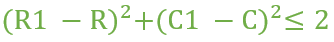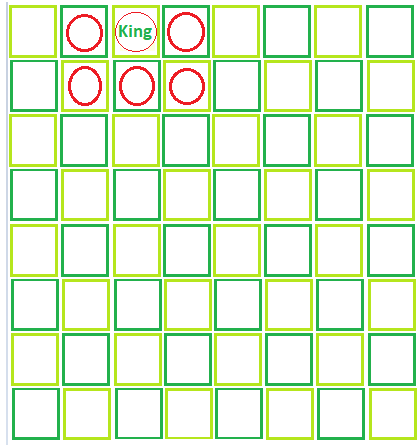# Total position where king can reach on a chessboard in exactly M moves

Given an integer M, an 8 * 8 chessboard and the king is placed on one of the square of the chessboard. Let the coordinate of the king be (R, C).
Note that the king can move to a square whose coordinate is (R1, C1) if and only if below condition is satisfied.The task is to count the number of position where the king can reach (excluding the initial position) from the given square in exactly M moves.

Examples:

Input: row = 1, column = 3, moves = 1
Output: Total number of position where king can reached = 5Input: row = 2, column = 5, moves = 2
Output: Total number of position where king can reached = 19

## Recommended: Please try your approach on {IDE} first, before moving on to the solution.

Approach: Calculate the coordinates of the top left square that can be visited by the king (a, b) and the coordinates of the bottom right square (c, d) of the chessboard that the king can visit. Then the total number of cells that the king can visit will be (c – a + 1) * (d – b + 1) – 1.

Below is the implementation of the above approach:

## C++

 `// C++ implementation of above approach ` `#include ` `using` `namespace` `std; ` ` `  `// Function to return the number of squares that ` `// the king can reach in the given number of moves ` `int` `Square(``int` `row, ``int` `column, ``int` `moves) ` `{ ` `    ``int` `a = 0, b = 0, c = 0, d = 0, total = 0; ` ` `  `    ``// Calculate initial and final coordinates ` `    ``a = row - moves; ` `    ``b = row + moves; ` `    ``c = column - moves; ` `    ``d = column + moves; ` ` `  `    ``// Since chessboard is of size 8X8 so if ` `    ``// any coordinate is less than 1 or greater than 8 ` `    ``// make it 1 or 8. ` `    ``if` `(a < 1) ` `        ``a = 1; ` `    ``if` `(c < 1) ` `        ``c = 1; ` `    ``if` `(b > 8) ` `        ``b = 8; ` `    ``if` `(d > 8) ` `        ``d = 8; ` ` `  `    ``// Calculate total positions ` `    ``total = (b - a + 1) * (d - c + 1) - 1; ` `    ``return` `total; ` `} ` ` `  `// Driver code ` `int` `main() ` `{ ` `    ``int` `R = 4, C = 5, M = 2; ` `    ``cout << Square(R, C, M); ` ` `  `    ``return` `0; ` `} `

## Java

 `// Java implementation of above approach ` `class` `GFG ` `{ ` ` `  `// Function to return the number  ` `// of squares that the king can ` `// reach in the given number of moves ` `static` `int` `Square(``int` `row, ``int` `column, ` `                            ``int` `moves) ` `{ ` `    ``int` `a = ``0``, b = ``0``, c = ``0``, ` `        ``d = ``0``, total = ``0``; ` ` `  `    ``// Calculate initial and final coordinates ` `    ``a = row - moves; ` `    ``b = row + moves; ` `    ``c = column - moves; ` `    ``d = column + moves; ` ` `  `    ``// Since chessboard is of size 8X8  ` `    ``// so if any coordinate is less  ` `    ``// than 1 or greater than 8 make ` `    ``// it 1 or 8. ` `    ``if` `(a < ``1``) ` `        ``a = ``1``; ` `    ``if` `(c < ``1``) ` `        ``c = ``1``; ` `    ``if` `(b > ``8``) ` `        ``b = ``8``; ` `    ``if` `(d > ``8``) ` `        ``d = ``8``; ` ` `  `    ``// Calculate total positions ` `    ``total = (b - a + ``1``) * (d - c + ``1``) - ``1``; ` `    ``return` `total; ` `} ` ` `  `// Driver code ` `public` `static` `void` `main(String []args) ` `{ ` `    ``int` `R = ``4``, C = ``5``, M = ``2``; ` `    ``System.out.println(Square(R, C, M)); ` `} ` `} ` ` `  `// This code is contributed by Ita_c. `

## Python3

 `# Python3 implementation of above approach  ` ` `  `# Function to return the number of  ` `# squares that the king can reach  ` `# in the given number of moves  ` `def` `Square(row, column, moves) : ` `     `  `    ``a ``=` `0``; b ``=` `0``; c ``=` `0``;  ` `    ``d ``=` `0``; total ``=` `0``;  ` ` `  `    ``# Calculate initial and final  ` `    ``# coordinates  ` `    ``a ``=` `row ``-` `moves;  ` `    ``b ``=` `row ``+` `moves;  ` `    ``c ``=` `column ``-` `moves;  ` `    ``d ``=` `column ``+` `moves;  ` ` `  `    ``# Since chessboard is of size 8X8  ` `    ``# so if any coordinate is less than  ` `    ``# 1 or greater than 8 make it 1 or 8.  ` `    ``if` `(a < ``1``) : ` `        ``a ``=` `1``;  ` `    ``if` `(c < ``1``) :  ` `        ``c ``=` `1``;  ` `    ``if` `(b > ``8``) : ` `        ``b ``=` `8``;  ` `    ``if` `(d > ``8``) : ` `        ``d ``=` `8``;  ` ` `  `    ``# Calculate total positions  ` `    ``total ``=` `(b ``-` `a ``+` `1``) ``*` `(d ``-` `c ``+` `1``) ``-` `1``;  ` `    ``return` `total;  ` ` `  `# Driver code  ` `if` `__name__ ``=``=` `"__main__"` `: ` ` `  `    ``R ``=` `4``; C ``=` `5``; M ``=` `2``;  ` `    ``print``(Square(R, C, M));  ` `     `  `# This code is contributed by Ryuga `

## C#

 `// C# implementation of above approach ` `using` `System; ` ` `  `class` `GFG ` `{ ` ` `  `// Function to return the number  ` `// of squares that the king can ` `// reach in the given number of moves ` `static` `int` `Square(``int` `row, ``int` `column, ` `                            ``int` `moves) ` `{ ` `    ``int` `a = 0, b = 0, c = 0, ` `        ``d = 0, total = 0; ` ` `  `    ``// Calculate initial and final coordinates ` `    ``a = row - moves; ` `    ``b = row + moves; ` `    ``c = column - moves; ` `    ``d = column + moves; ` ` `  `    ``// Since chessboard is of size 8X8  ` `    ``// so if any coordinate is less  ` `    ``// than 1 or greater than 8 make ` `    ``// it 1 or 8. ` `    ``if` `(a < 1) ` `        ``a = 1; ` `    ``if` `(c < 1) ` `        ``c = 1; ` `    ``if` `(b > 8) ` `        ``b = 8; ` `    ``if` `(d > 8) ` `        ``d = 8; ` ` `  `    ``// Calculate total positions ` `    ``total = (b - a + 1) * (d - c + 1) - 1; ` `    ``return` `total; ` `} ` ` `  `// Driver code ` `public` `static` `void` `Main() ` `{ ` `    ``int` `R = 4, C = 5, M = 2; ` `    ``Console.Write(Square(R, C, M)); ` `} ` `} ` ` `  `// this code is contributed by Ita_c. `

## PHP

 ` 8) ` `        ``\$b` `= 8; ` `    ``if` `(``\$d` `> 8) ` `        ``\$d` `= 8; ` ` `  `    ``// Calculate total positions ` `    ``\$total` `= (``\$b` `- ``\$a` `+ 1) *  ` `             ``(``\$d` `- ``\$c` `+ 1) - 1; ` `    ``return` `\$total``; ` `} ` ` `  `// Driver code ` `\$R` `= 4; ``\$C` `= 5; ``\$M` `= 2; ` `echo` `Square(``\$R``, ``\$C``, ``\$M``); ` ` `  `// This code is contributed  ` `// by Akanksha Rai ` `?> `

Output:

```24
```My Personal Notes arrow_drop_upCheck out this Author's contributed articles.

If you like GeeksforGeeks and would like to contribute, you can also write an article using contribute.geeksforgeeks.org or mail your article to contribute@geeksforgeeks.org. See your article appearing on the GeeksforGeeks main page and help other Geeks.

Please Improve this article if you find anything incorrect by clicking on the "Improve Article" button below.